## Eccentric AnomalyThe Angle obtained by drawing the Auxiliary Circle of an Ellipse with center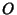and Focus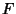, and drawing a Line Perpendicular to the Semimajor Axis and intersecting it at. The Angle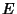is then defined as illustrated above. Then for an Ellipse with Eccentricity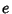,(1)

But the distance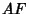is also given in terms of the distance from the Focusand the Supplement of the Angle from the Semimajor Axis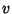by(2)

Equating these two expressions gives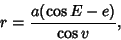(3)

which can be solved for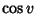to obtain(4)

To getin terms of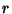, plug (4) into the equation of the Ellipse(5)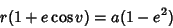(6)(7)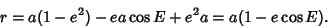(8)

Differentiating gives(9)

The eccentric anomaly is a very useful concept in orbital mechanics,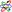where it is related to the so-called mean anomalyby Kepler's Equation(10)can also be interpreted as the Area of the shaded region in the above figure (Finch).

Finch, S. Favorite Mathematical Constants.'' http://www.mathsoft.com/asolve/constant/lpc/lpc.html